Publicité

# Lesson 1.2 NT (Equation and Inequalities).pdf

23 Mar 2023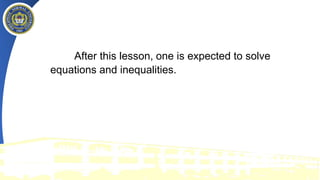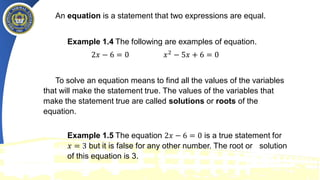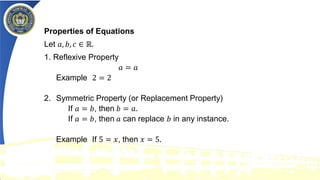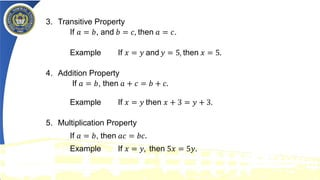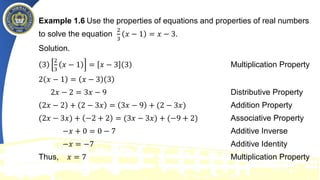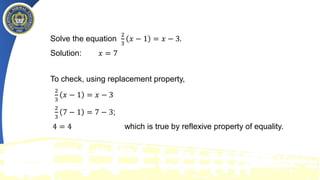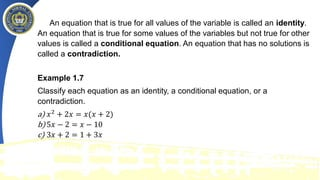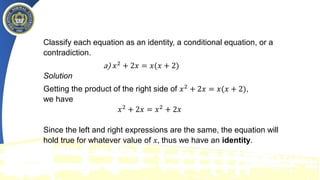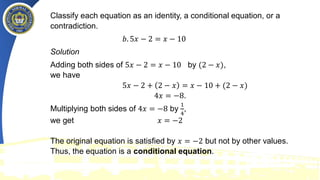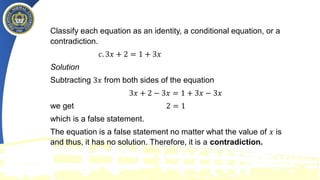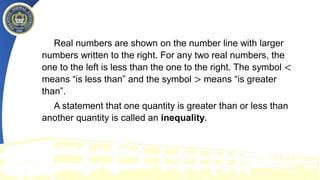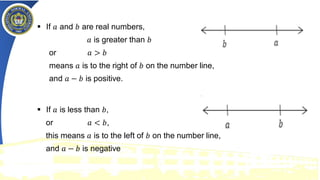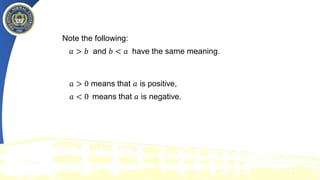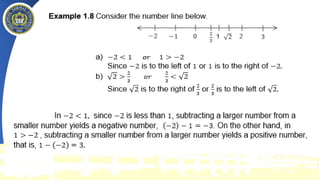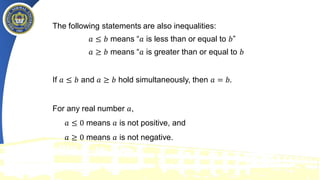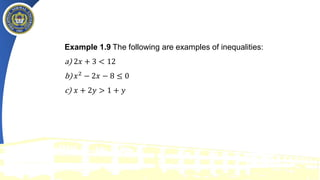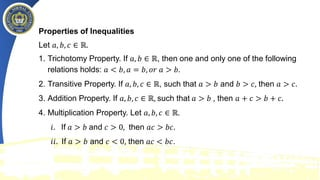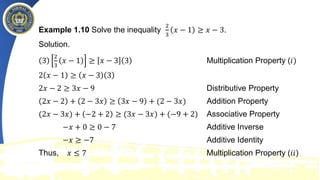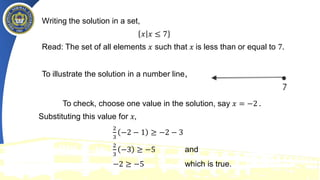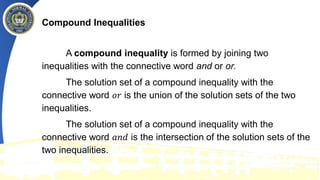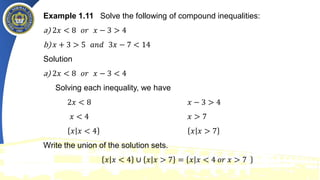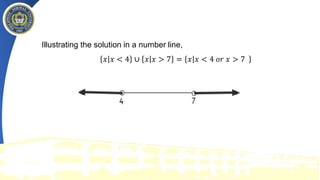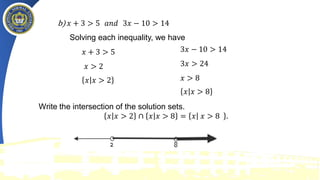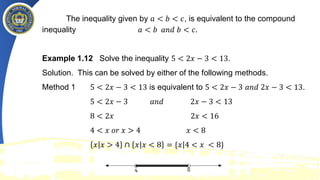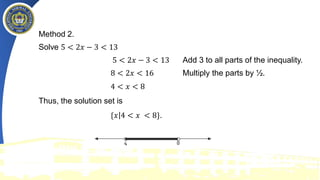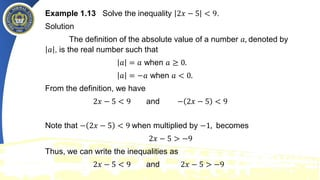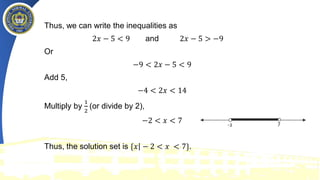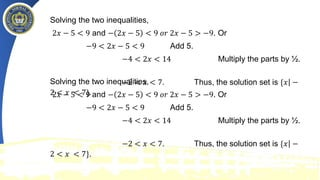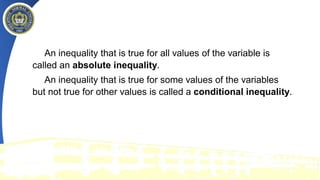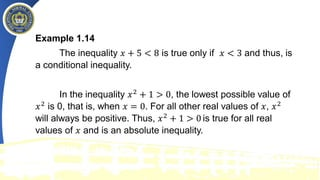1 sur 32
Publicité

### Lesson 1.2 NT (Equation and Inequalities).pdf

1. PHILIPPINE NORMAL UNIVERSITY VISAYAS The National Center for Teacher Education The Environment and Green Technology Education Hub Cadiz City, Negros Occidental
2. PHILIPPINE NORMAL UNIVERSITY VISAYAS The National Center for Teacher Education The Environment and Green Technology Education Hub Cadiz City, Negros Occidental
3. After this lesson, one is expected to solve equations and inequalities.
4. An equation is a statement that two expressions are equal. Example 1.4 The following are examples of equation. 2𝑥 − 6 = 0 𝑥2 − 5𝑥 + 6 = 0 To solve an equation means to find all the values of the variables that will make the statement true. The values of the variables that make the statement true are called solutions or roots of the equation. Example 1.5 The equation 2𝑥 − 6 = 0 is a true statement for 𝑥 = 3 but it is false for any other number. The root or solution of this equation is 3.
5. Properties of Equations Let 𝑎, 𝑏, 𝑐 ∈ ℝ. 1. Reflexive Property 𝑎 = 𝑎 Example 2 = 2 2. Symmetric Property (or Replacement Property) If 𝑎 = 𝑏, then 𝑏 = 𝑎. If 𝑎 = 𝑏, then 𝑎 can replace 𝑏 in any instance. Example If 5 = 𝑥, then 𝑥 = 5.
6. 3. Transitive Property If 𝑎 = 𝑏, and 𝑏 = 𝑐, then 𝑎 = 𝑐. Example If 𝑥 = 𝑦 and 𝑦 = 5, then 𝑥 = 5. 4. Addition Property If 𝑎 = 𝑏, then 𝑎 + 𝑐 = 𝑏 + 𝑐. Example If 𝑥 = 𝑦 then 𝑥 + 3 = 𝑦 + 3. 5. Multiplication Property If 𝑎 = 𝑏, then 𝑎𝑐 = 𝑏𝑐. Example If 𝑥 = 𝑦, then 5𝑥 = 5𝑦.
7. Example 1.6 Use the properties of equations and properties of real numbers to solve the equation 2 3 𝑥 − 1 = 𝑥 − 3. Solution. 3 2 3 𝑥 − 1 = 𝑥 − 3 3 Multiplication Property 2 𝑥 − 1 = 𝑥 − 3 3 2𝑥 − 2 = 3𝑥 − 9 Distributive Property 2𝑥 − 2 + 2 − 3𝑥 = 3𝑥 − 9 + (2 − 3𝑥) Addition Property (2𝑥 − 3𝑥) + −2 + 2 = (3𝑥 − 3𝑥) + (−9 + 2) Associative Property −𝑥 + 0 = 0 − 7 Additive Inverse −𝑥 = −7 Additive Identity Thus, 𝑥 = 7 Multiplication Property
8. Solve the equation 2 3 𝑥 − 1 = 𝑥 − 3. Solution: 𝑥 = 7 To check, using replacement property, 2 3 𝑥 − 1 = 𝑥 − 3 2 3 7 − 1 = 7 − 3; 4 = 4 which is true by reflexive property of equality.
9. An equation that is true for all values of the variable is called an identity. An equation that is true for some values of the variables but not true for other values is called a conditional equation. An equation that has no solutions is called a contradiction. Example 1.7 Classify each equation as an identity, a conditional equation, or a contradiction. a) 𝑥2 + 2𝑥 = 𝑥(𝑥 + 2) b) 5𝑥 − 2 = 𝑥 − 10 c) 3𝑥 + 2 = 1 + 3𝑥
10. Classify each equation as an identity, a conditional equation, or a contradiction. a) 𝑥2 + 2𝑥 = 𝑥(𝑥 + 2) Solution Getting the product of the right side of 𝑥2 + 2𝑥 = 𝑥(𝑥 + 2), we have 𝑥2 + 2𝑥 = 𝑥2 + 2𝑥 Since the left and right expressions are the same, the equation will hold true for whatever value of 𝑥, thus we have an identity.
11. Classify each equation as an identity, a conditional equation, or a contradiction. 𝑏. 5𝑥 − 2 = 𝑥 − 10 Solution Adding both sides of 5𝑥 − 2 = 𝑥 − 10 by (2 − 𝑥), we have 5𝑥 − 2 + 2 − 𝑥 = 𝑥 − 10 + (2 − 𝑥) 4𝑥 = −8. Multiplying both sides of 4𝑥 = −8 by 1 4 , we get 𝑥 = −2 The original equation is satisfied by 𝑥 = −2 but not by other values. Thus, the equation is a conditional equation.
12. Classify each equation as an identity, a conditional equation, or a contradiction. 𝑐. 3𝑥 + 2 = 1 + 3𝑥 Solution Subtracting 3𝑥 from both sides of the equation 3𝑥 + 2 − 3𝑥 = 1 + 3𝑥 − 3𝑥 we get 2 = 1 which is a false statement. The equation is a false statement no matter what the value of 𝑥 is and thus, it has no solution. Therefore, it is a contradiction.
13. Real numbers are shown on the number line with larger numbers written to the right. For any two real numbers, the one to the left is less than the one to the right. The symbol < means “is less than” and the symbol > means “is greater than”. A statement that one quantity is greater than or less than another quantity is called an inequality.
14. ▪ If 𝑎 and 𝑏 are real numbers, 𝑎 is greater than 𝑏 or 𝑎 > 𝑏 means 𝑎 is to the right of 𝑏 on the number line, and 𝑎 − 𝑏 is positive. ▪ If 𝑎 is less than 𝑏, or 𝑎 < 𝑏, this means 𝑎 is to the left of 𝑏 on the number line, and 𝑎 − 𝑏 is negative
15. Note the following: 𝑎 > 𝑏 and 𝑏 < 𝑎 have the same meaning. 𝑎 > 0 means that 𝑎 is positive, 𝑎 < 0 means that 𝑎 is negative.
16. The following statements are also inequalities: 𝑎 ≤ 𝑏 means “𝑎 is less than or equal to 𝑏” 𝑎 ≥ 𝑏 means “𝑎 is greater than or equal to 𝑏 If 𝑎 ≤ 𝑏 and 𝑎 ≥ 𝑏 hold simultaneously, then 𝑎 = 𝑏. For any real number 𝑎, 𝑎 ≤ 0 means 𝑎 is not positive, and 𝑎 ≥ 0 means 𝑎 is not negative.
17. Example 1.9 The following are examples of inequalities: a) 2𝑥 + 3 < 12 b) 𝑥2 − 2𝑥 − 8 ≤ 0 c) 𝑥 + 2𝑦 > 1 + 𝑦
18. Properties of Inequalities Let 𝑎, 𝑏, 𝑐 ∈ ℝ. 1. Trichotomy Property. If 𝑎, 𝑏 ∈ ℝ, then one and only one of the following relations holds: 𝑎 < 𝑏, 𝑎 = 𝑏, 𝑜𝑟 𝑎 > 𝑏. 2. Transitive Property. If 𝑎, 𝑏, 𝑐 ∈ ℝ, such that 𝑎 > 𝑏 and 𝑏 > 𝑐, then 𝑎 > 𝑐. 3. Addition Property. If 𝑎, 𝑏, 𝑐 ∈ ℝ, such that 𝑎 > 𝑏 , then 𝑎 + 𝑐 > 𝑏 + 𝑐. 4. Multiplication Property. Let 𝑎, 𝑏, 𝑐 ∈ ℝ. 𝑖. If 𝑎 > 𝑏 and 𝑐 > 0, then 𝑎𝑐 > 𝑏𝑐. 𝑖𝑖. If 𝑎 > 𝑏 and 𝑐 < 0, then 𝑎𝑐 < 𝑏𝑐.
19. Example 1.10 Solve the inequality 2 3 𝑥 − 1 ≥ 𝑥 − 3. Solution. 3 2 3 𝑥 − 1 ≥ 𝑥 − 3 3 Multiplication Property (𝑖) 2 𝑥 − 1 ≥ 𝑥 − 3 3 2𝑥 − 2 ≥ 3𝑥 − 9 Distributive Property 2𝑥 − 2 + 2 − 3𝑥 ≥ 3𝑥 − 9 + (2 − 3𝑥) Addition Property (2𝑥 − 3𝑥) + −2 + 2 ≥ (3𝑥 − 3𝑥) + (−9 + 2) Associative Property −𝑥 + 0 ≥ 0 − 7 Additive Inverse −𝑥 ≥ −7 Additive Identity Thus, 𝑥 ≤ 7 Multiplication Property (𝑖𝑖)
20. Writing the solution in a set, 𝑥ȁ𝑥 ≤ 7 Read: The set of all elements 𝑥 such that 𝑥 is less than or equal to 7. To illustrate the solution in a number line, To check, choose one value in the solution, say 𝑥 = −2 . Substituting this value for 𝑥, 2 3 −2 − 1 ≥ −2 − 3 2 3 −3 ≥ −5 and −2 ≥ −5 which is true.
21. Compound Inequalities A compound inequality is formed by joining two inequalities with the connective word and or or. The solution set of a compound inequality with the connective word 𝑜𝑟 is the union of the solution sets of the two inequalities. The solution set of a compound inequality with the connective word 𝑎𝑛𝑑 is the intersection of the solution sets of the two inequalities.
22. Example 1.11 Solve the following of compound inequalities: a) 2𝑥 < 8 𝑜𝑟 𝑥 − 3 > 4 b) 𝑥 + 3 > 5 𝑎𝑛𝑑 3𝑥 − 7 < 14 Solution a) 2𝑥 < 8 𝑜𝑟 𝑥 − 3 < 4 Solving each inequality, we have 2𝑥 < 8 𝑥 − 3 > 4 𝑥 < 4 𝑥 > 7 𝑥ȁ𝑥 < 4 𝑥ȁ𝑥 > 7 Write the union of the solution sets. 𝑥ȁ𝑥 < 4 ∪ 𝑥ȁ𝑥 > 7 = 𝑥ȁ𝑥 < 4 𝑜𝑟 𝑥 > 7
23. Illustrating the solution in a number line, 𝑥ȁ𝑥 < 4 ∪ 𝑥ȁ𝑥 > 7 = 𝑥ȁ𝑥 < 4 𝑜𝑟 𝑥 > 7
24. b) 𝑥 + 3 > 5 𝑎𝑛𝑑 3𝑥 − 10 > 14 Solving each inequality, we have 𝑥 + 3 > 5 𝑥 > 2 𝑥ȁ𝑥 > 2 3𝑥 − 10 > 14 3𝑥 > 24 𝑥 > 8 𝑥ȁ𝑥 > 8 Write the intersection of the solution sets. 𝑥ȁ𝑥 > 2 ∩ 𝑥ȁ𝑥 > 8 = 𝑥ȁ 𝑥 > 8 .
25. The inequality given by 𝑎 < 𝑏 < 𝑐, is equivalent to the compound inequality 𝑎 < 𝑏 𝑎𝑛𝑑 𝑏 < 𝑐. Example 1.12 Solve the inequality 5 < 2𝑥 − 3 < 13. Solution. This can be solved by either of the following methods. Method 1 5 < 2𝑥 − 3 < 13 is equivalent to 5 < 2𝑥 − 3 𝑎𝑛𝑑 2𝑥 − 3 < 13. 5 < 2𝑥 − 3 𝑎𝑛𝑑 2𝑥 − 3 < 13 8 < 2𝑥 2𝑥 < 16 4 < 𝑥 𝑜𝑟 𝑥 > 4 𝑥 < 8 𝑥ȁ𝑥 > 4 ∩ ȁ 𝑥 𝑥 < 8 = { ȁ 𝑥 4 < 𝑥 < 8}
26. Method 2. Solve 5 < 2𝑥 − 3 < 13 5 < 2𝑥 − 3 < 13 Add 3 to all parts of the inequality. 8 < 2𝑥 < 16 Multiply the parts by ½. 4 < 𝑥 < 8 Thus, the solution set is { ȁ 𝑥 4 < 𝑥 < 8}.
27. Example 1.13 Solve the inequality 2𝑥 − 5 < 9. Solution The definition of the absolute value of a number 𝑎, denoted by 𝑎 , is the real number such that 𝑎 = 𝑎 when 𝑎 ≥ 0. 𝑎 = −𝑎 when 𝑎 < 0. From the definition, we have 2𝑥 − 5 < 9 and − 2𝑥 − 5 < 9 Note that − 2𝑥 − 5 < 9 when multiplied by −1, becomes 2𝑥 − 5 > −9 Thus, we can write the inequalities as 2𝑥 − 5 < 9 and 2𝑥 − 5 > −9
28. Thus, we can write the inequalities as 2𝑥 − 5 < 9 and 2𝑥 − 5 > −9 Or −9 < 2𝑥 − 5 < 9 Add 5, −4 < 2𝑥 < 14 Multiply by 1 2 (or divide by 2), −2 < 𝑥 < 7 Thus, the solution set is { ȁ 𝑥 − 2 < 𝑥 < 7}.
29. Solving the two inequalities, 2𝑥 − 5 < 9 and − 2𝑥 − 5 < 9 𝑜𝑟 2𝑥 − 5 > −9. Or −9 < 2𝑥 − 5 < 9 Add 5. −4 < 2𝑥 < 14 Multiply the parts by ½. −2 < 𝑥 < 7. Thus, the solution set is { ȁ 𝑥 − 2 < 𝑥 < 7}. Solving the two inequalities, 2𝑥 − 5 < 9 and − 2𝑥 − 5 < 9 𝑜𝑟 2𝑥 − 5 > −9. Or −9 < 2𝑥 − 5 < 9 Add 5. −4 < 2𝑥 < 14 Multiply the parts by ½. −2 < 𝑥 < 7. Thus, the solution set is { ȁ 𝑥 − 2 < 𝑥 < 7}.
30. An inequality that is true for all values of the variable is called an absolute inequality. An inequality that is true for some values of the variables but not true for other values is called a conditional inequality.
31. Example 1.14 The inequality 𝑥 + 5 < 8 is true only if 𝑥 < 3 and thus, is a conditional inequality. In the inequality 𝑥2 + 1 > 0, the lowest possible value of 𝑥2 is 0, that is, when 𝑥 = 0. For all other real values of 𝑥, 𝑥2 will always be positive. Thus, 𝑥2 + 1 > 0 is true for all real values of 𝑥 and is an absolute inequality.
Publicité